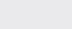Course Content

0/1

0/3

0/5

0/3

0/2

0/4

0/2

0/1

0/5

5.4 Hyperbola 双曲线

(1)

Find the centre, vertex, axis of symmetry, transverse semi-axis, conjugate semi-axis, eccentricity, focus, directrix, length of latus rectum and asymptotes for the following hyperbola and sketch the graph.

(a) 9x– y= 9

(b) y– 4x= 1

(c) 4x– 9y– 16x – 18y – 29 = 0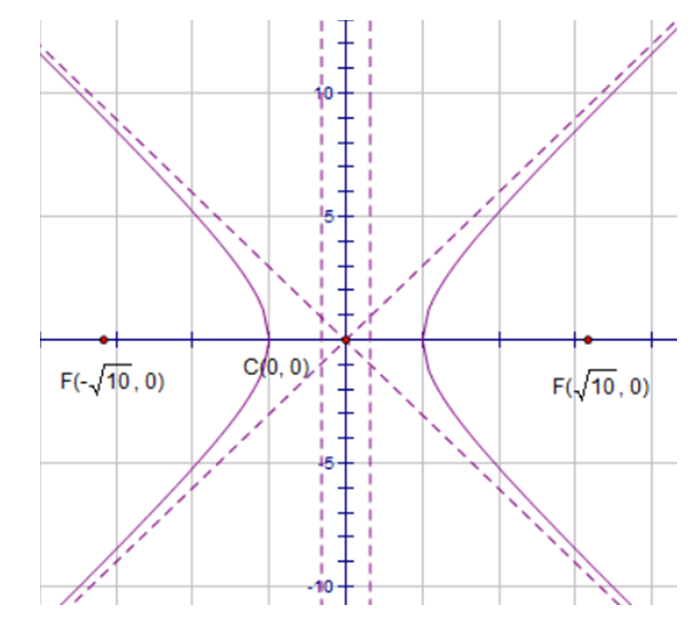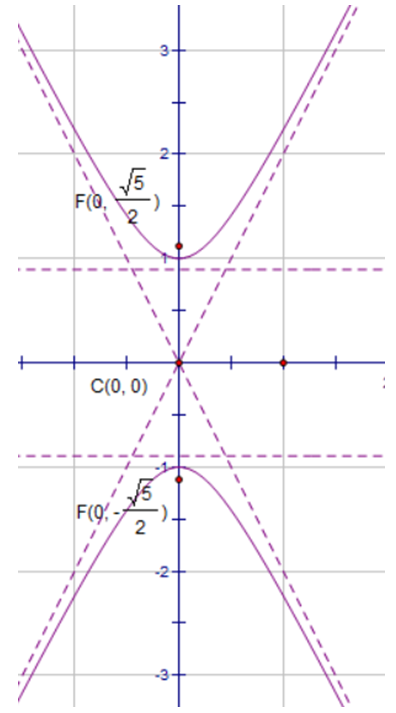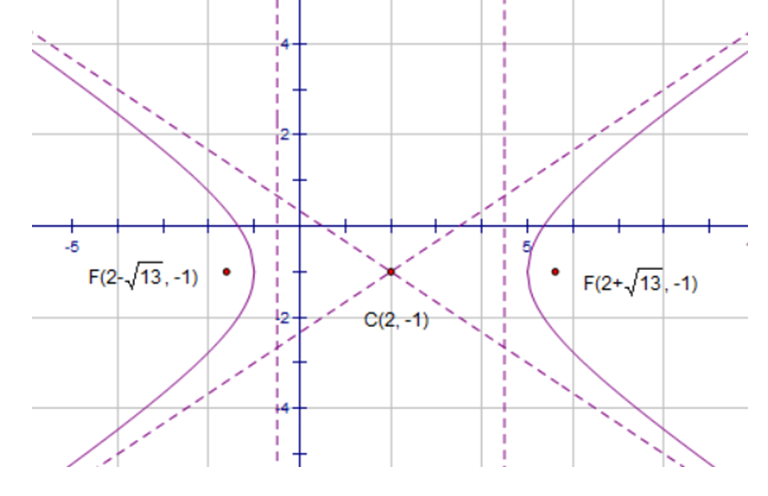(2)

Find the equation of the hyperbola with center at (-7, -2), transverse axis parallel to the x-axis, eccentricity, latus rectum.(3)

Find the equation of the hyperbola with asymptotes 2x – y = 0, 2x + y – 4 = 0 and passes through the point (6, 10).

(2x – y) (2x + y – 4) = 36
(4)

Find the equation of the hyperbolic with center (3, -6), conjugate axis parallel to the x-axis, distance between foci, distance between directrices.(5)

Given that P is on the right side of the hyperbola, its distance to the right directrix is, calculate the distance of P to the left focus.

11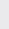If the hyperbolaand circledon’t have intersection point, find the range of the real value k.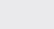or